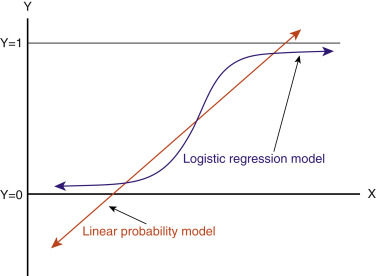03/10/2023

# Logistic RegressionMachine Learning (ML) algorithms are using in the science world from health to agriculture in every area and about all researches for new innovations. Every opportunity that I have I try to give you a brief and concise explanation about these algorithms in our web platform majorscope.com

In this article, we will explore one of the coolest ML models, and glance at its implementations in the data science world.  It is Logistic Regression.

Logistic regression, despite its name, is a linear model for classification rather than regression. Logistic regression is also known in the literature as logit regression, maximum-entropy classification (MaxEnt) or the log-linear classifier. In this model, the probabilities describing the possible outcomes of a single trial are modeled using a

We won’t go to the further deep analysis about model’s formulas. You can always check detailed functions and formulas in the scikit-learn web portal. Instead, we try to figure out how it works in practical researches.First of all Logistic Regression is a method for classification practice.  Although the name may be confusing at first, logistic regression allows us to solve classification problems, where we are trying to predict discrete categories.  Unlike linear regression problems where we try to predict a continuous value. This model focus on Binary Classifications, have two classes 0 and 1, like;

• Spam versus “Ham” emails
• Loan Default (yes/no)
• Disease Diagnosis(malignant/benign)

We can’t use a normal linear regression model for binary groups. Instead, we can transform our linear regression to a logistic regression curve(as shown above chart). We use the logistic function to output a value ranging from 0 to 1. Based off of this probability we assign a class. (That means we change Linear Regression Solution into the Sigmoid Function.)The luxury steamship RMS Titanic sank in the early hours of April 15, 1912, off the coast of Newfoundland in the North Atlantic after sideswiping an iceberg during its maiden voyage. Of the 2,240 passengers and crew on board, more than 1,500 lost their lives in the disaster.https://www.history.com/topics/early-20th-century-us/titanic  jQuery('#footnote_plugin_tooltip_1523_2_2').tooltip({ tip: '#footnote_plugin_tooltip_text_1523_2_2', tipClass: 'footnote_tooltip', effect: 'fade', predelay: 0, fadeInSpeed: 200, delay: 400, fadeOutSpeed: 200, position: 'top center', relative: true, offset: [-7, 0], });

We practice this binary model for the Titanic data set. You can download the data from references. In this project the data set has been split into two groups:

• training set (train.csv)
• test set (test.csv)

The training set is used to build our machine learning model (logistic regression).  Our model is based on “features” like passengers’ gender and class. The test set is used to see how well our model performs on unseen data. You may get more information at the data reference.

Step by step we build our model. Respectively we have gone through some processes; first we imported the necessary libraries, and loaded the data then we made Exploratory Data Analysis based on this analysis, we cleaned the Data, did some intuitive feature engineering, after all these processes lastly we trained our model and predicted test results. You can reach all of the analysis for the model at the link depicted in references.

Our goal is to predict the passengers whether they were survived or not survived based on the logistic regression model. After we trained a logistic regression model on titanic training data, we evaluated our model’s performance on target test data, and the results are great.

Our model has an estimated 81 percent accuracy. It is a great success to reach this score by analyzing the limited data at hand.  We can also use a confusion matrix to evaluate the classification models. Our models’ confusion matrix results are as in the below chart;As per our model chart, 216 (148+68) times predicted true and 41 (36+15) times false. We achieved these results with a logistic regression model, you can try other ML models and can get better results.

Briefly to say; at the dawn of the machine learning, in every science area, many models such as logistic regression, using the binary classification model, are light attitudes to research in all subjects. As in the example we examined, we can easily analyze a historical event by means of machine learning and clearly, the closed areas of history can be illuminated. You may use artificial intelligence and machine learning models and tools to facilitate the world of science and your own life.
See you in the next article.

### Author

This site uses Akismet to reduce spam. Learn how your comment data is processed.